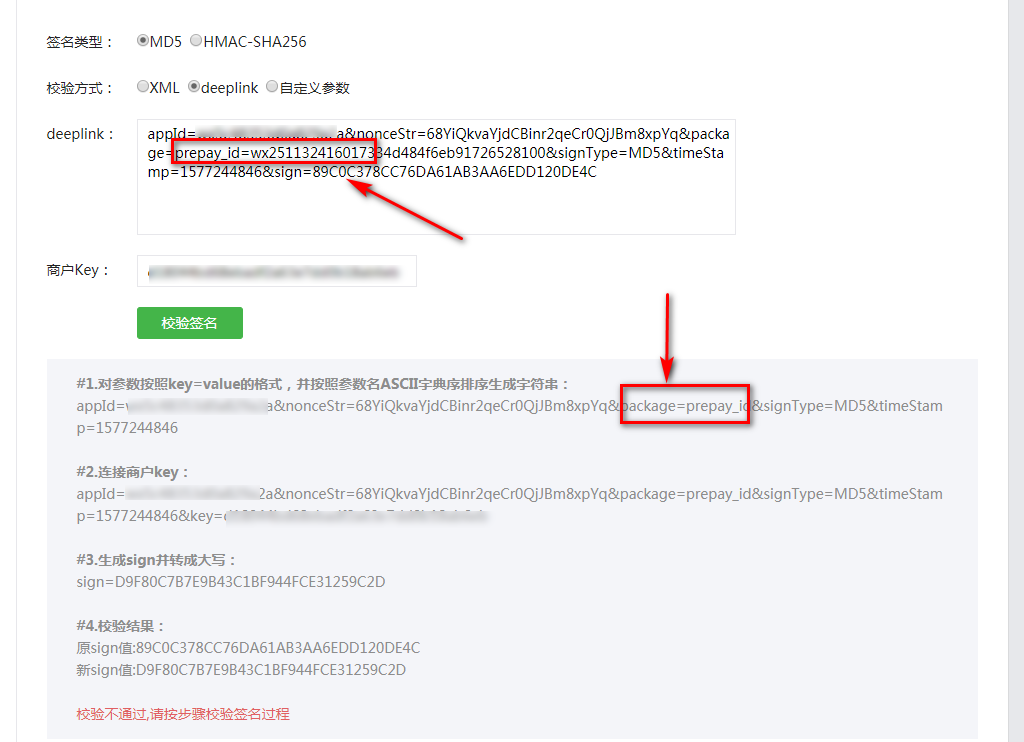# 置顶paySign签名遇到的问题,packaged 在做 签名时 = 号后面值不会被计算到签名中区精选热门

//- > 前端代码

"appId":data.appId,     //公众号名称，由商户传入

"timeStamp":data.timeStamp,         //时间戳，自1970年以来的秒数

"nonceStr":data.nonceStr, //随机串

"package":data.packageValue,    //-> 这里我是 使用   "prepay_id="+prepayId; 得到的,package是关键字,所以需要处理

"signType":data.signType,         //微信签名方式：

"paySign":data.paySign //微信签名

//-> java 代码

StringBuilder signValue = new StringBuilder(200);

//-> 公众号名称

signValue.append("appId").append('=').append(this.appId).append('&');

//-> 随机数

signValue.append("nonceStr").append('=').append(Utils.random(32)).append('&');

//-> this.packageValue = "prepay_id="+prepayId ; 所谓的 package

signValue.append("package").append('=').append(this.packageValue).append('&');

//-> MD5

signValue.append("signType").append('=').append("MD5").append('&');

//-> 当前秒

signValue.append("timeStamp").append('=').append(WXPayUtil.getCurrentTimestamp()).append('&');

//-> 秘钥值

signValue.append("key=").append(Token.KEY);

System.err.println(signValue);

this.sign = WXPayUtil.MD5(signValue.toString());

//->这是计算签名的代码,

//-> 计算过后得到的值 然后去微信 那边比对 是不一致的 就是微信提供的测试工具//-> data里面的值是能保证 一致的 我怀疑的是 微信js做签名的时候 吧 = 后的值 移除了 所以计算的签名不一致, 使用 %3D 然而又找不到 total_fee

2 个回答